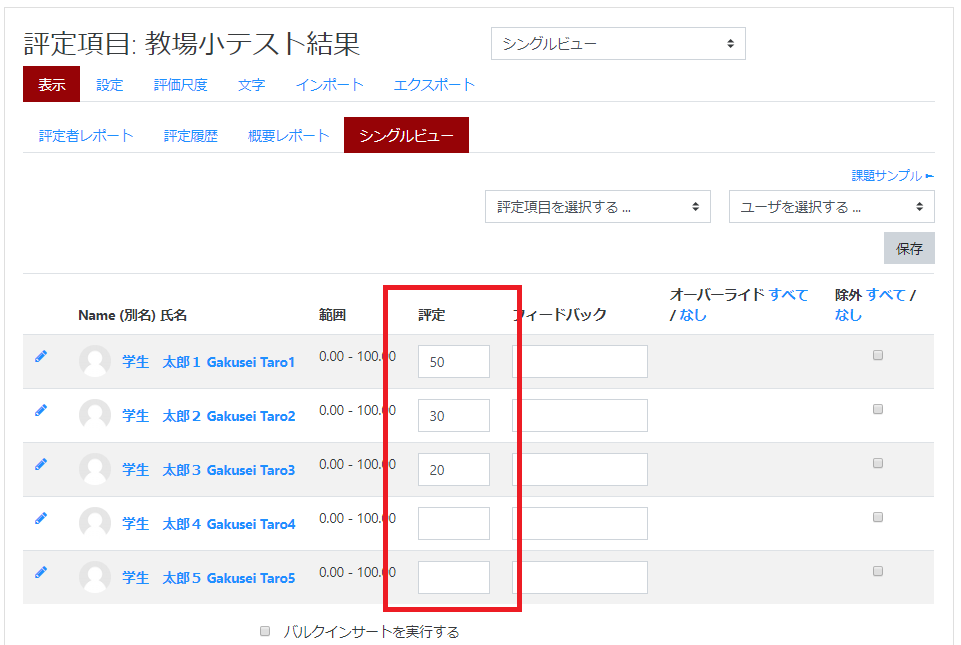In the setting of grades, you can set weights for grades of each content in “Gradebook setup.”

##### Weights setting

You can set weights by each content.
The default value is “0.0”, which will not be reflected in the “Course Total” at this point. If you set it to “1” for all content and save it, it will all have the same specific gravity and will be reflected in the “Course Total” based on the grade of each content.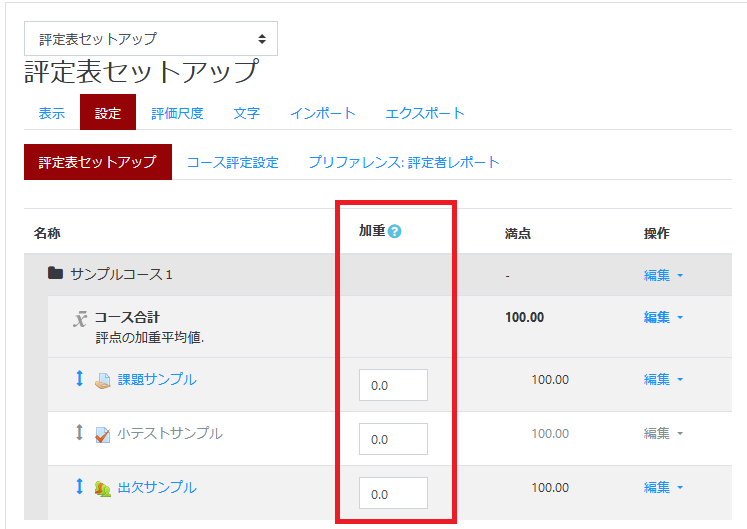In the following example, the assignment and quiz grades are twice as heavy as the attendance grades.
In the “Weight” column, enter “Assignment sample” = 2, “Quiz sample” = 2, “Attendance sample” = 1, and click [Save changes].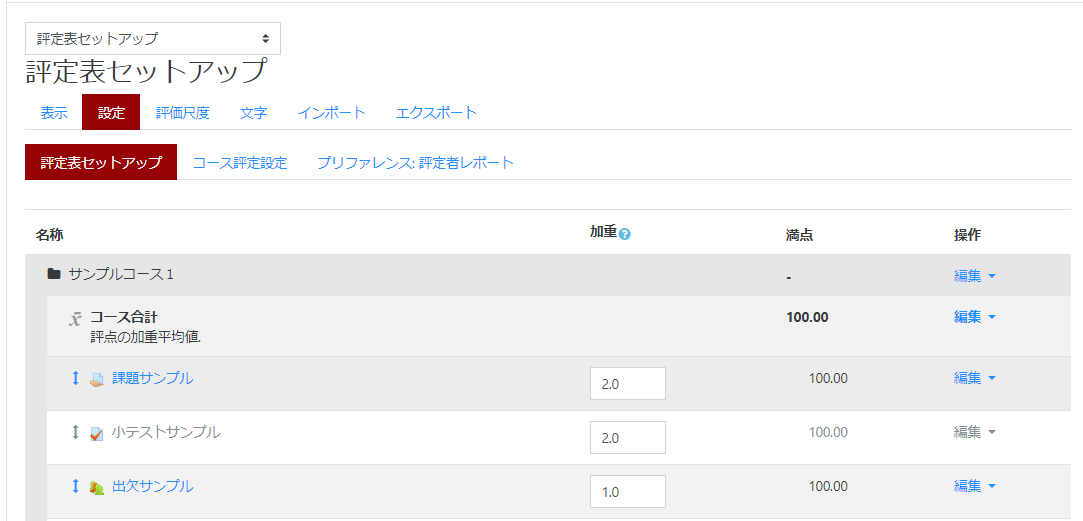When the grader report is displayed, the value of the total grade of the course (converted to 100 points) changes according to the weight. For example, it is assumed that “Student Taro 1” got “Assignment sample” = 100 points, “Quiz sample” = 80 points, “Attendance sample” = 60 points.
Then, Assignment sample : Quiz sample : Attendance sample = 2:2:1,
Thus 40 points (100 *2/5) + 32 points (80*2/5) + 12 points (60*1/5) = 84 points is the grade of the entire course.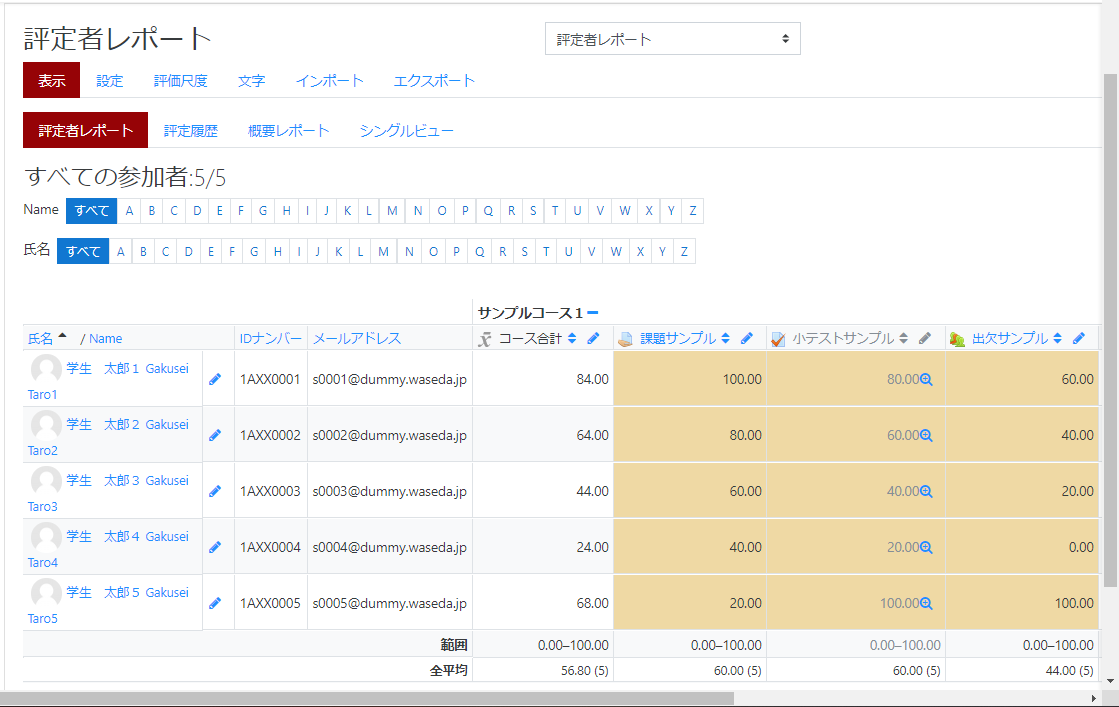memo
Only if each piece of content has a “grade” in it, will it be included in the formula for calculating the course total. Therefore, be sure to enter a grade of “0” or higher for each piece of content you want to include in the grading formulas, and include a grade of Please note that if you leave a “-” mark in the assignment, it will not be included in the calculation. If you do not submit the assignment and leave it at “-“, it will not be included in the calculation(*).
Specifically, Student Taro 2 has a score of 40 on the “Teaching Field Quiz Results” = “40” and “Assignment for Lecture 3” = “60”. Let’s say that you have scored a “score” and “Lecture 3 quiz” = “80 points”. At this time, if the weighting is set to 1.0 for all three contents, the result of the quiz will be: lecture 3 Assignment: 10 points (30 points*1/3) + 20 points (60) because the quiz in Lecture 3 = 1:1:1 Points*1/3) + 30 points (90 points*1/3) = 60 points for the entire course.
On the other hand, “Student Taro 4” has a “teaching field quiz result” = “90” and “Lecture 3 assignment” = “-” (not yet Suppose you have a “Lecture 3 quiz” = “60 points” (not to be graded by submission).
At this time, if the weighting is set to 1.0 for all three contents, the result of the quiz will be: “Lecture 3 quiz = 1:1: (Lecture 3 assignments are not graded), so the score is 45 (90 points*1/ 2) + 30 points (60 points*1/2) = 75 points for the entire course. If the student has not submitted the “Lecture 3” assignment and the grade is “0”, then 30 points (90 points*1/3) + 0 Points (0*1/3) + 20 points (60*1/3) = 50 points for the entire course (“The (Student Taro 3 is in this state).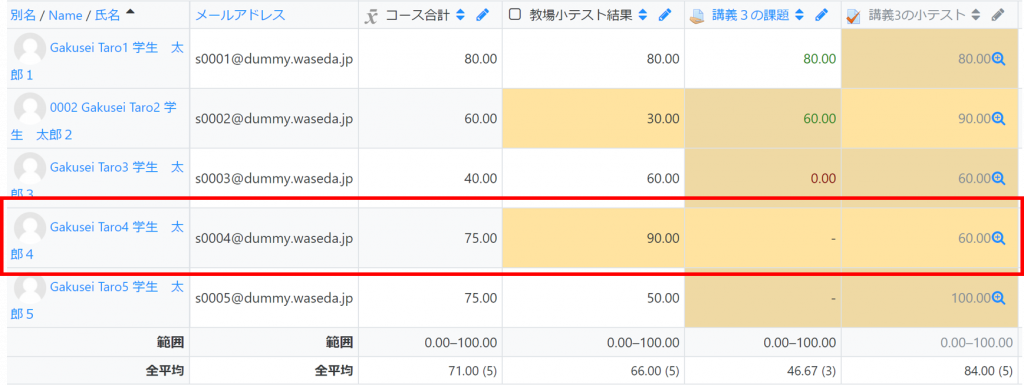*If you want to include content with a grade of “-” in the calculation, you need to change the settings.
See “Changes to Assessment Scope” below.
##### Changes to Assessment Scope

If you want to include content with a “grade” of “-” (blank grade) in the calculation, click “Setup” in the Grader Report.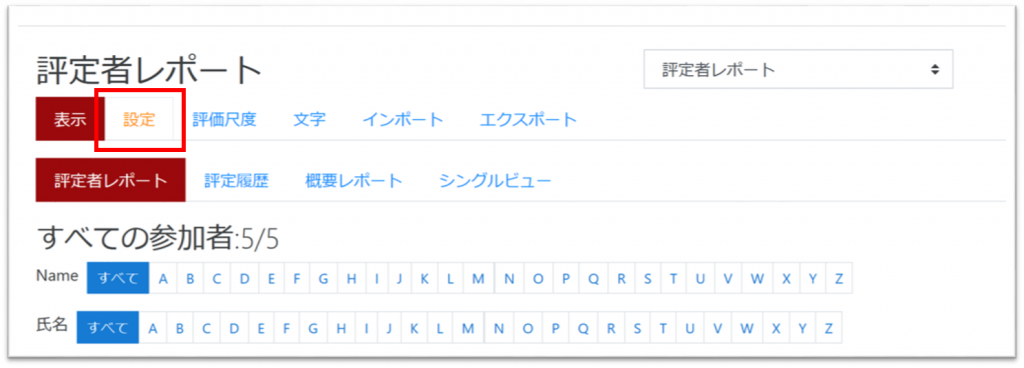Click the “Edit” button to the right of the subject name, and then click “Edit Settings”.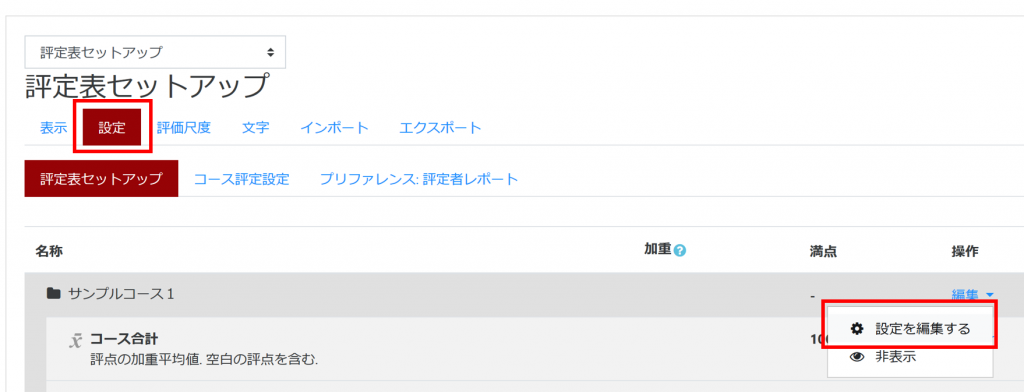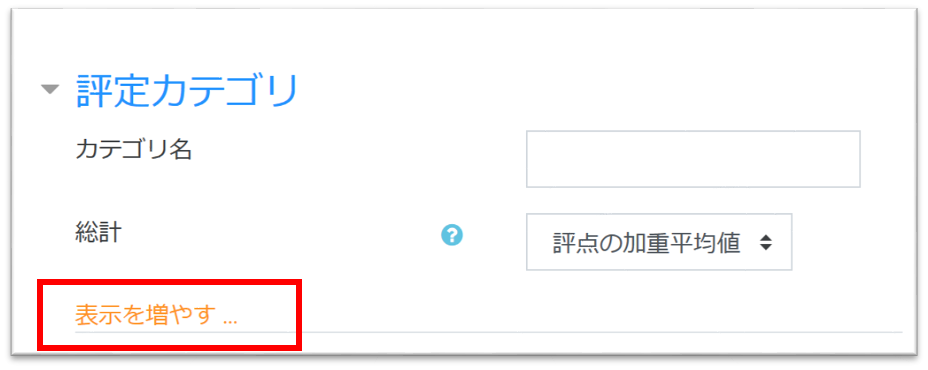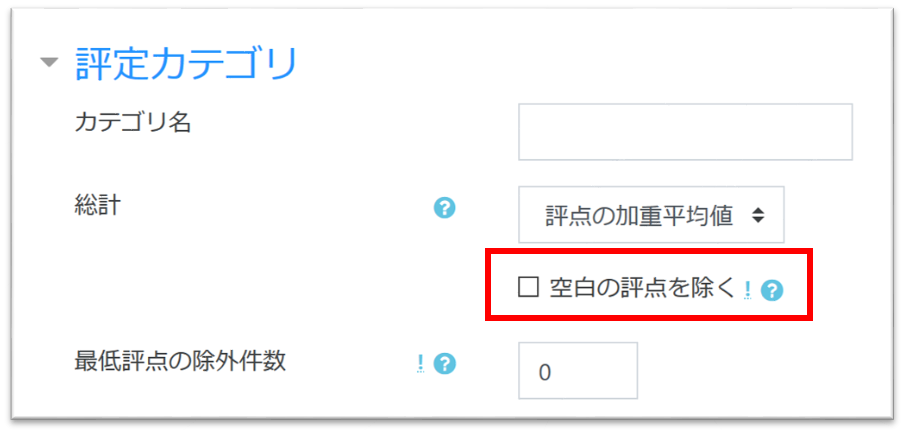It will recalculate and when it is done, click “Continue” to complete the process.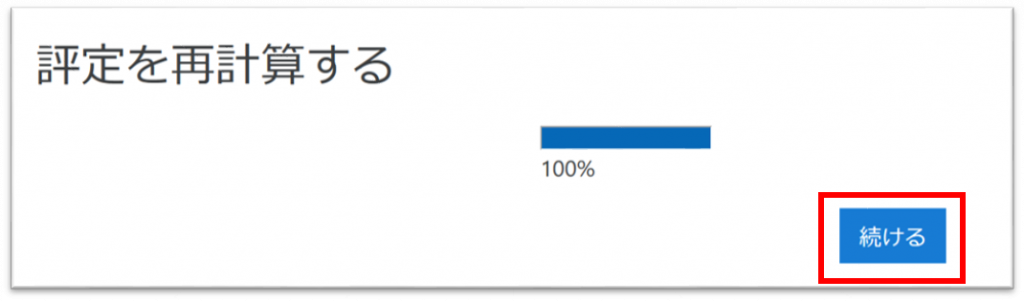The course total is calculated for both Gakusei Taro 3 and Gakusei Taro 4, including contents that are marked “-” (blank grades).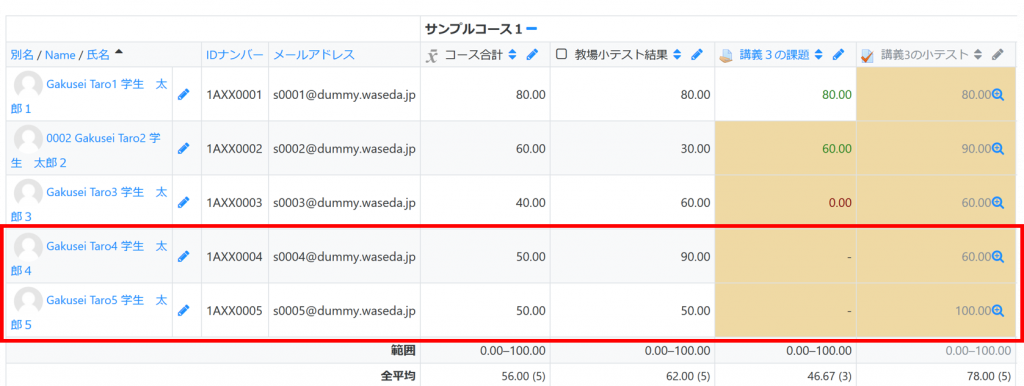memo
By default, a “-” (blank grade) is set to “0”.
If you want to change the value for the “-” (blank grades), go to Grades, Setup, Edit, Edit settings, Category total, click on “Show more” and then change the “Minimum Grade”.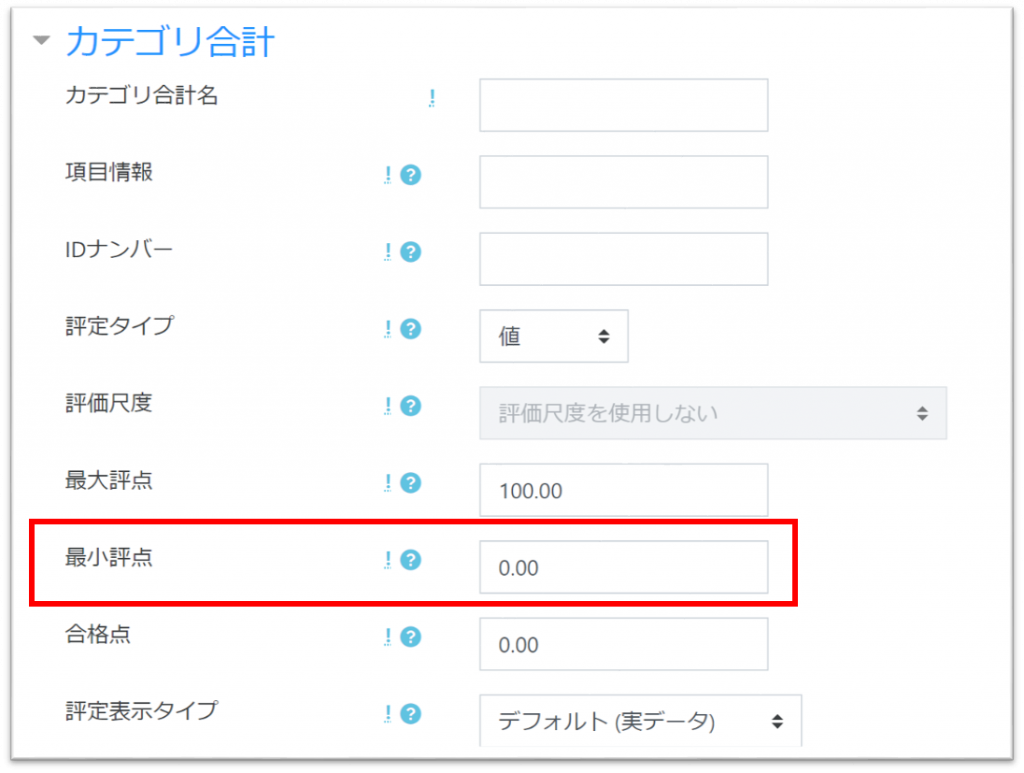You can import data that does not exist in Waseda Moodle, such as the results of class room quiz.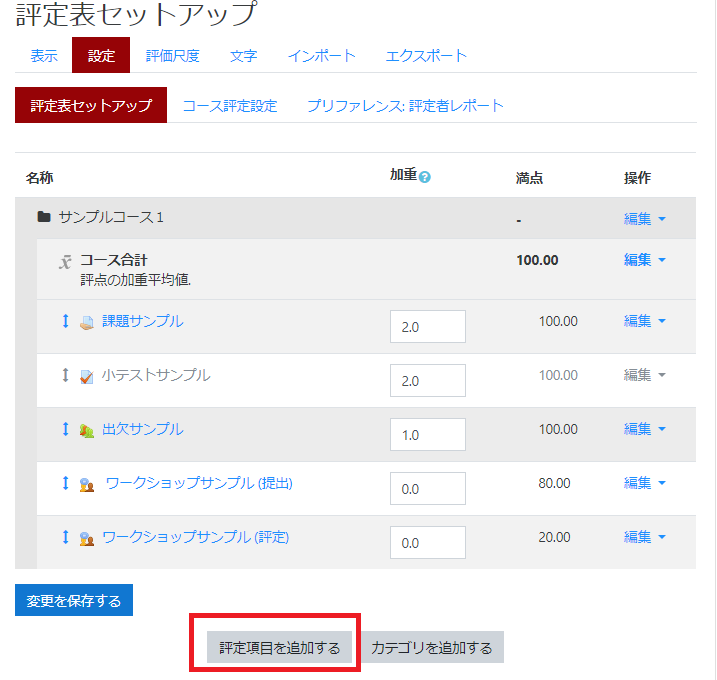Enter “Item name” and click [Save changes].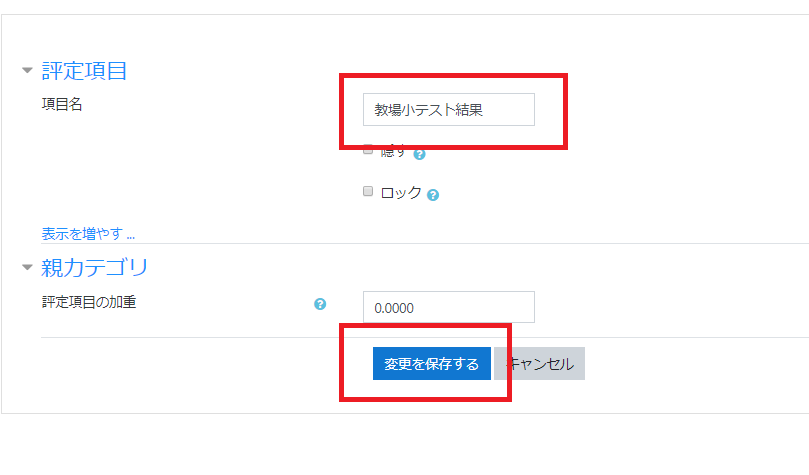The grade items added to “Grader report” will be displayed. For the example, “Classroom quiz” is displayed.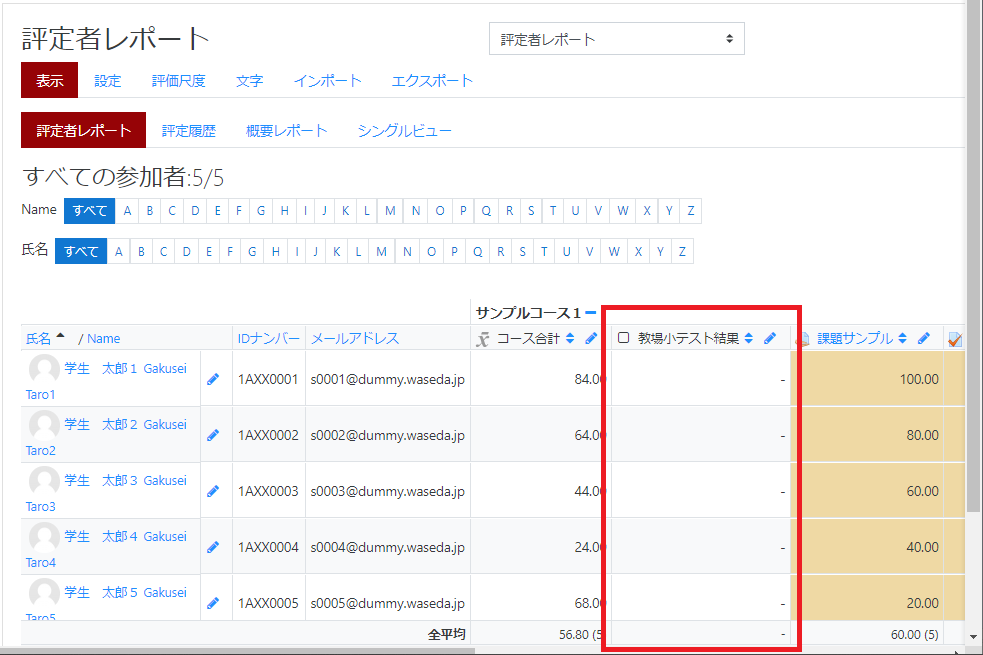You can enter grades from [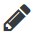] icon.